# Bihar Board 12th Maths Model Question Paper 2 in English Medium

Bihar Board 12th Maths Model Papers

## Bihar Board 12th Maths Model Question Paper 2 in English Medium

Time : 3 Hours 15 Min
Full Marks: 100

Instructions for the candidates :

1. Candidates are required to give their answers in their own words as far as practicable.
2. Figure in the right-hand margin indicates full marks.
3. While answering the questions, the candidate should adhere to the word limit as far as practicable.
4. 15 Minutes of extra time has been allotted for the candidate to read the questions carefully.
5. This question paper is divided into two sections. Section-A and Section-B
6. In Section A, there are 1-50 objective type questions which are compulsory, each carrying 1 mark. Darken the circle with blue/black ball pen against the correct option on the OMR Sheet provided to you. Do not use Whitener/Liquid/ Blade/Nail on OMR Sheet otherwise result will be invalid.
7. In section-B, there are 25 short answer type questions (each carrying 2 marks), out of which only 15 (fifteen) questions are to be answered.
A part from this there is 08 Long Answer Type questions (each carrying 5 marks), out of which 4 questions are to be answered.
8. Use of any electronic device is prohibited.

Objective Type Questions

There are 1 to 50 objective type questions with 4 options, choose the correct option which is to be answered on OMR Sheet (50 × 1 = 50)

Question 1.
Let R be the relation in the set {1, 2, 3, 4} given by R = {(1,2), (2,2), (1,1), (4,4), (1,3), (3,3), (3,2)}.
(a) R is reflexive and symmetric but not transitive
(b) R is reflexive and transitive but not symmetric.
(c) R is symmetric and transitive but not reflexive
(d) R is an equivalence relation
(b) R is reflexive and transitive but not symmetric.Question 2.
The number of binary operation in set {a, b} is
(a) 10
(b) 16
(c) 20
(d) 8
(b) 16

Question 3.
The range of $$f(x)=\sqrt{(x-1)(3-x)}$$ is
(a) [1,3]
(b) [0,1]
(c) [ -2,2 ]
(d) None
(b) [0,1]Question 4.
$$2 \tan ^{-1} \frac{1}{3}+\tan ^{-1} \frac{1}{7}=$$
(a) $$\tan ^{-1} \frac{44}{29}$$
(b) $$\frac{\pi}{2}$$
(c) 0
(d) $$\frac{\pi}{4}$$
(d) $$\frac{\pi}{4}$$

Question 5.
$$\cos ^{-1}\left(\cos \frac{7 \pi}{6}\right)$$ =
(a) $$\frac{7 \pi}{6}$$
(b) $$\frac{\pi}{3}$$
(c) $$\frac{\pi}{6}$$
(d) $$\frac{5 \pi}{6}$$
(d) $$\frac{5 \pi}{6}$$

Question 6.
sin-1 (1 – x) – 2sin -1x = $$\frac{\pi}{2}$$ so x = :
(a) 0,$$\frac{1}{2}$$
(b) 1,$$\frac{1}{2}$$
(c) $$\frac{1}{2}$$
(d) 0
(a) 0,$$\frac{1}{2}$$

Question 7.
If sin-1 x + sin-1 y = $$\frac{\pi}{3}$$ then cos-1 x + cos-1 y is equal to
(a) $$\frac{\pi}{6}$$
(b) $$\frac{\pi}{3}$$
(c) $$\frac{2\pi}{3}$$
(d) π
(c) $$\frac{2\pi}{3}$$

Question 8.
$$\int \frac{k^{\sqrt{x}}}{\sqrt{x}} d x=$$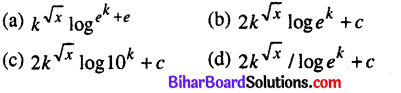$$2 k^{\sqrt{x}} / \log e^{k}+c$$

Question 9.
$$\int \frac{1}{\sin x+\cos x} d x=$$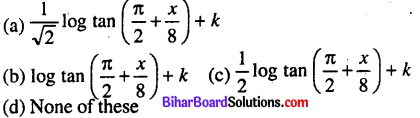$$\frac{1}{\sqrt{2}} \log \tan \left(\frac{\pi}{2}+\frac{x}{8}\right)+k$$Question 10.
$$\frac{d}{d x} \int f(x) d x=$$
(a) f'(x)
(b) f(x)
(c) f'(x)
(d) f(x) + c
(b) f(x)

Question 11.
$$\int_{0}^{1} \frac{f(x)}{f(x)+f(1-x)} d x=$$
(a) 0
(b) 1/2
(c) 1
(d) None
(b) 1/2

Question 12.
$$\int_{-\pi / 2}^{\pi / 2} \sin ^{2} x d x=$$
(a) 0
(b) $$\frac{\pi}{2}$$
(c) 1
(d) π
(a) 0

Question 13.
$$\int_{0}^{\pi}(\sqrt{\tan x}+\sqrt{\cot x}) d x=$$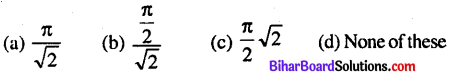$$\frac{\pi}{\sqrt{2}}$$

Question 14.
$$\int \frac{1}{x^{\frac{1}{3}}} d x=$$(a) $$\frac{3}{2} x^{2 / 3}+c$$

Question 15.
$$\frac{\int_{-\pi}^{\pi}\left(x^{3}+x \cos x+\tan ^{5} x+1\right) d x}{2}$$ =
(a) $$\frac{\pi}{2}$$
(b) π
(c) 0
(d) 2
(b) πQuestion 16.
If x>a, then $$\int \frac{d x}{x^{2}-a^{2}} d x=$$$$\frac{1}{2 a} \log \frac{x-a}{x+a}+k$$

Question 17.
The total revenue in Rupees received from the sale of x units of a product is given by R (x) = 13x2 + 26x +15.
Then marginal revenue when x = 7 is
(a) Rs. 208.
(b) Rs. 308
(c) Rs. 140
(d) Rs. 508
(a) Rs. 208.

Question 18.
In what interval the function log x is strictly increasing.
(a) (∞,0)
(b) (-∞,0)
(c) (0, ∞)
(d) (0,-∞)
(c) (0, ∞)

Question 19.
The slope of normal to the curve y = 2x2 + 3 sin x at the point x = 0 is
(a) 3
(b) 1/3
(c) -3
(d) -1/3
(d) -1/3

Question 20.
$$\int_{-\frac{\pi}{2}}^{\pi / 2} \sin ^{7} x d x=?$$
(a) 1
(b) -1
(c) 0
(d) None
(c) 0Question 21.
$$\int \frac{1}{x} d x=?$$
(a) loge1/x
(b) logex + c
(c) logax + c
(d) None of these
(b) logex + c

Question 22.
∫tan x dx = ?
(a) log cos x + c
(b) log sec x + c
(c) log cot x + c
(d) None of these
(b) log sec x + c

Question 23.
The value of $$\int_{0}^{1} e^{x} d x$$ is
(a) e – 1
(b) e
(c) 1
(d) None
(a)e – 1

Question 24.
If f(-x)=-f(x) then which of the value of $$\int_{-a}^{a} f(x) d x$$
(a) f(a)
(b) 2f(a)
(c) 0
(d) None
(c) 0

Question 25.
$$\int \frac{1+x}{x^{2}} d x=?$$(a) $$\log x-\frac{1}{x}+c$$

Question 26.
The area of the region enclosed by y2 = 4ax and latus rectum is(b) $$\frac{8 a_{\cdot}^{2}}{3} \text { unit }^{2}$$

Question 27.
Let f(x) = $$\int_{1}^{\pi} \sqrt{2-t^{2}} d t$$ then real root of the equation x2 -f'(x) = 0 is
(a) $$\pm \frac{1}{2}$$
(b) ±1
(c) $$\pm \frac{1}{\sqrt{2}}$$
(d) ±2
(b) ±1Question 28.
The order of diff.eqn $$\left(\frac{d \cdot^{2} y}{d x^{2}}\right)^{4}+3\left(\frac{d y}{d x}\right) \cdot^{3}+9 y=\cos x$$ is
(a) 4
(b) 3
(c) 2
(d) None
(c) 2

Question 29.
The degree of diff. eqn $$\left\{1+\cdot\left(\frac{d y}{d x}\right) \cdot^{2}\right\}^{3 / 2}=m \frac{d^{2} y}{d x^{2}}$$
(a) 2
(b) 3
(c) 1
(d) 0
(c) 1

Question 30.
The order of diff. eqn $$2 x^{2} \frac{d^{2} y}{d x^{2}}-3 \frac{d y}{d x}+y=0)$$
(a) 2
(b) 1
(c) 0
(d) Not defined
(a) 2

Question 31.
The integrating factor of diff. eqn $$x \frac{d y}{d x}-y=2 x^{2}$$ is
(a) e-x
(b) e-y
(c) 1/x
(d) x
(a) e-xQuestion 32.
The number of arbitrary constants in the particular solution of the differential equation of order third is
(a) 3
(b) 2
(c) 1
(d) 0
(a) 3

Question 33.
Which of the statement is true for ∆ABC:(c) $$\overrightarrow{A B}+\overrightarrow{B C}-\overrightarrow{C A}=0$$

Question 34.
Let $$\vec{a}$$ and $$\vec{b}$$ be two unit vectors and 6 is the angle – between them. Then $$\vec{a}+\vec{b}$$ is unit vector if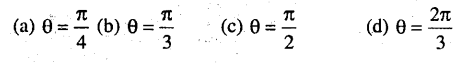(d) $$\theta=\frac{2 \pi}{3}$$

Question 35.
The value of $$\hat{i} \cdot(\hat{j} \times \hat{k})+\hat{j} \cdot(\hat{i} \times \hat{k})+\hat{k} \cdot(\hat{i} \times \hat{j})$$ is
(a) 0
(b) -1
(c) 1
(d) 3
(c) 1Question 36.
The position vector of the three points are $$\vec{a}-2 \vec{b}+3 \vec{c}, 2 \vec{a}+3 \vec{b}-4 \vec{c}, 7 \vec{b}-10 \vec{c}$$. Then Points are
(a) Collinear
(b) Coplanar
(c) Non-coplanar
(d) None of these
(a) Collinear

Question 37.
If a line makes the angle α, β and γ with the x,y and Z-axes then
(a) cos2 α + cos2 β + cos2 γ = -1
(b) sin2 α + sin2 β + sin2 γ = 2
(c) cos2 α+cos2 β + cos2 γ = 2
(d) cos2 α + cos2 β + cos2 γ = 1
(b) sin2 α + sin2 β + sin2 γ = 2

Question 38.
If x-axis makes angle 0°, 90°, 90° with the x, y and Z-axes respectively. Then direction cosine of x-axis is
(a) 0,0,1
(b) 1,0,0
(c) 0,0,1
(d) 0,1,0
(b) 1,0,0

Question 39.
If the direction cosines of two lines are lv mv nl and l1, m12,n1 and l2, m2, n2 respectively, then mutually perpendicular if
(a) $$\frac{l_{1}}{l_{2}}=\frac{m_{1}}{m_{2}}=\frac{n_{1}}{n_{2}}]$$
(b) l1l2 + m1m2 + n1n2 = 0
(c) l1m1n1 + l2m2n2
(d) None of these
l1l2 + m1m2 + n1n2 = 0

Question 40.
The distance of the plane 2x-3y + 4z-6 = 0 from the origin is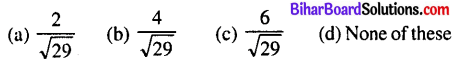(c) $$\frac{6}{\sqrt{29}}$$

Question 41.
If the plane has the intercepts a,b,c and is at a distance of p units from the origin is(b) $$\frac{1}{a^{2}}+\frac{1}{b^{2}}+\frac{1}{c^{2}}=\frac{1}{p^{2}}g$$Question 42.
The feasible region due to non-negative constraints will lie in which of the following quadrants ?
(a) Fourth
(b) First
(c) Third
(d) Second
(b) First

Question 43.
The graph of linear equation ax + by = c, which is a straight line will passes through which of the following point on the x-axis is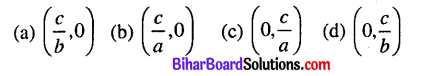(b) $$\left(\frac{c}{a}, 0\right)$$

Question 44.
If A and Bare two events such that $$\mathbf{P}\left(\frac{\mathbf{A}}{\mathbf{B}}\right)=\mathbf{P}\left(\frac{\mathbf{B}}{\mathbf{A}}\right) \neq \mathbf{0}$$ Then
(a)A ⊆ B
(b) A = B
(c) A ∩ B = Φ
(d) P(A) = P(B)
(d) P(A) = P(B)

Question 45.
If P(A) = $$\frac{7}{13}$$ ,P(B) = $$\frac{9}{13}$$ and P(A ∩B)= $$\frac{4}{13}$$ . Then P($$\frac{A}{B}$$) is equal to
(a) $$\frac{3}{9}$$
(b) $$\frac{4}{9}$$
(c) $$\frac{5}{19}$$
(d) $$\frac{7}{9}$$
(b) $$\frac{4}{9}$$

Question 46.
If P($$\frac{A}{B}$$) > P(A) Then which of the following is true ?
(a) P (B/A) < P(B)
(b) P (A ∩B) < P(A).P(B) (c) P (B/A) > P(B)
(d) P (B/A) = P(B)
(c) P (B/A) > P(B)

Question 47.
Which of the following is probability distribution.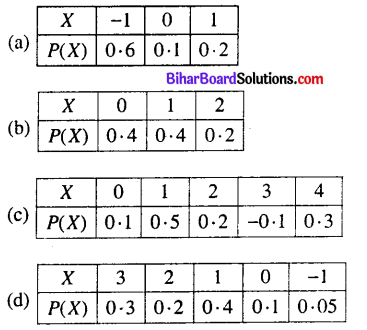Question 48.
If A and B are any two events such that P(A) = 0.2, P(B) = 0.6. Then P(A∪B)+P(A∩B) = ?
(a) 0.4
(b) 0.8
(c) 0.12
(d) 0.9
(b) 0.8

Question 49.
A dice is thrown 6 times. If “getting 6 success is”.
(a) $$\frac{1}{64}$$
(b) $$\frac{3}{32}$$
(c) $$\frac{7}{64}$$
(d) None of these
(a) $$\frac{1}{64}$$

Question 50.
If A and B are two mutually exclusive events such that p(A) = $$\frac{1}{6}$$ and P(B) = $$\frac{1}{2}$$ then which of the following is the value of P(A ∩ B).
(a) $$\frac{2}{3}$$
(b) 0
(c) $$\frac{1}{12}$$
(d) None
(c) $$\frac{1}{12}$$Non-Objective Type Questions

Q. No. 1 to 25 are of Short Answer type carry of 2 marks each. Answer any 15 questions. (15 × 2 = 30)

Question 1.
Let T be the set of all triangle in a plane define on R as {(T1, T2): T1 is congruence to T2} is an equivalence relation.
Let T1, T2, T3, ∈ T
(i) (T, T) ∈ R ⇒ TRT i.e. T is congruence to itself, so, R is reflexive.

(ii) (T1, T2) ∈ R ⇒ (T2, T1) ∈ R, T1 R T2 = T2 R T1, i.e. T1 congruence to T2 ⇒ T2 is congruence to T1.
So, R is symmetric

(iii) (T1,T2) ∈ R, (T2, T3) ∈ R ⇒ (T1,T3) ∈ R, T1 R T2T2 R T3 ⇒ T1 R T3 i.e. T1 congruence to T2, T2 is congruence to T3 ⇒ T1, is congnience to T3.
Since R is reflesive, symmetric and transitive, therefore R is an equivalence relation.Question 2.
If f: A → B 3ftr g: B → C be two functions one-one and onto then prove that (gof)-1 = f-1og-1
Since f: A → B and g: B → C two functions are one-one and onto, therefore /and g are invertible.
To show gof is invertible with (gof)-1 = f-1og-1, it is
enough to show that (f-1og-1) o (gof) = IA and (gof)o(f-1og-1) =IC.
Now, (f-1og-1) (gof) = ((f-1og-1)og)of
= (f-1o(g-1og)of = (f-1oIB) by definition of g-1 = IX …(1)
Similarly, it can be shown that
(gof)o(f-1og-1) = IC …(2)
From (1) and (2), we get (gof)-1 = f-1og-1

Question 3.
Prove that 4(cot-13 +cosec-1√5) = π
4(cot-13 + cosec-1√5) = π
Let cosec-1√5 = θ ⇒ cosecθ = √5
Take squaring, we get
cosec2 θ = 5 ⇒ 1 + cot2 θ= 5
⇒ cot2 θ = 5 – 1 = 4
⇒ cot2 θ = 4 ⇒ cot θ = 2
Tan θ = 1/2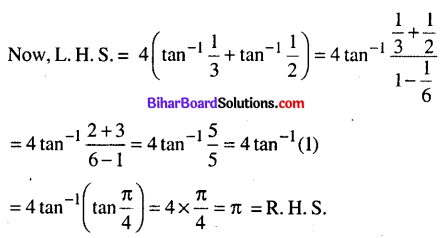Question 4.
For what value of x : $$\left[\begin{array}{lllll} 1 & 2 & 1 \end{array}\right]\left[\begin{array}{lll} 1 & 2 & 0 \\ 2 & 0 & 1 \\ 1 & 0 & 2 \end{array}\right]\left[\begin{array}{l} 0 \\ 2 \\ x \end{array}\right]=0$$Question 5.
Find the value of a, b, c, d if
$$\left[\begin{array}{cc} 2 a+b & a-2 b \\ 5 c-d & 4 c+3 d \end{array}\right]=\left[\begin{array}{cc} 4 & -3 \\ 11 & 24 \end{array}\right]$$
By equality of matrices
2a + b = 4 ……………… (1)
a-2b = -3 …(2) 5c – d = 11 ………..(3)
4c + 3d = 24 …………..(4)
Multiplying (1) by 2 and adding (2), we get
4a+2b + a-2b = 8-3 ⇒ 5a = 5⇒ a = 1
From(1), 2a + b = 4 ⇒ 2.1 + b = 4 ⇒ b = 4 – 2 = 2
Again, multiplying (3) by 3 and adding (4), we get
15c – 3J + 4c + 3d = 33 + 24 ⇒ 19c = 57 ⇒ c = 3
from(3), 5c – d = 11 ⇒ 5 × 3 – d = 11 ⇒ d = 15 – 11 = 4
Hence a = 1, b = 2, c = 3 and d = 4

Question 6.
If A =  then prove that |2A| = 4|A|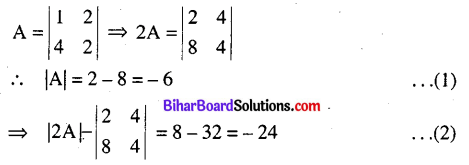From (1) and (2), we get |2A| = 4|A|Question 7.
Find the value of x by the equation :
$$\sin \left(\sin ^{-1} \frac{1}{5}+\cos ^{-1} x\right)=1$$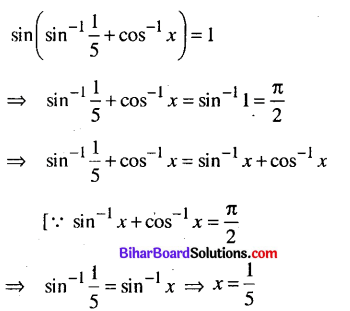Question 8.
If y = sin-1 then prove that (1 – x2)y2-xy1 = 0Question 9.
If f(x) =sin x/x when x ≠ 0, f(0) = 1. Then is fix) continuous at x = 0 ?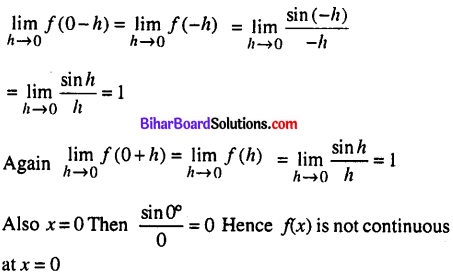Question 10.
Integrate : ∫secxdx
Multiplying Nr and Dr by sec x + tan x in integral, we get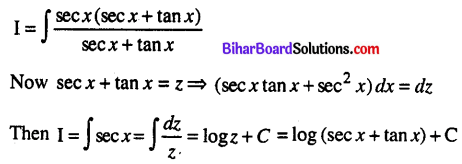Question 11.
Evaluate: $$\int_{0}^{\pi / 2} \frac{\sqrt{\sin x}}{\sqrt{\sin x}+\sqrt{\cos x}} d x$$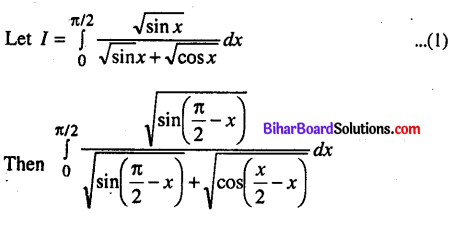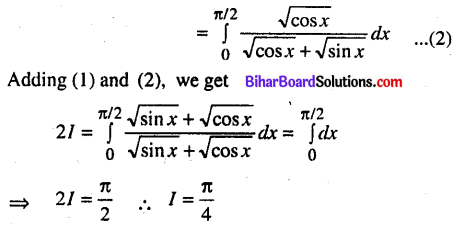Question 12.
Find $$\frac{d y}{d x}$$ for xy + 4 = 0at the point (-2,2)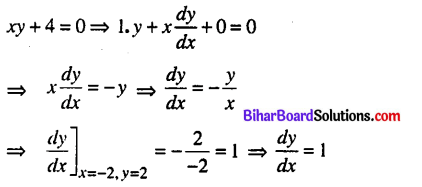Question 13.
Find the particular solution of the diff.eqn $$\frac{d y}{d x}$$= -4xy2 if y = 1 when x = 0.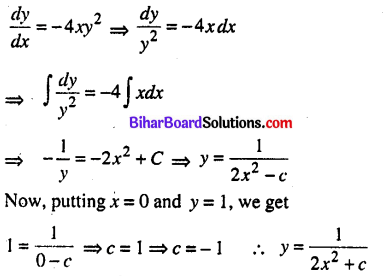Question 14.
Solve : (1 + x2)dy = (a + y2)dx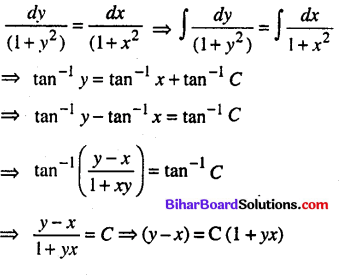Question 15.
If the vectors xî – 3ĵ + 5k̂ and -xî – xĵ + 2k̂ are mutually perpendicular then find the value of x.
Two vectors are perpendicular if $$\vec{a} \cdot \vec{b}=0$$
⇒ (xî – 3ĵ + 5k̂). (-xî + xĵ + 2k̂) = 0
⇒ -x2 – 3x +10 = 0 ⇒ x2 + 3x – 10 = 0
⇒ x2 – 5x + 2x – 10 = 0 ⇒ x(x – 5) + 2(x – 5) = 0
⇒ (x + 2) (x – 5) = 0 ⇒ x = -2,5

Question 16.
If $$\vec{a}$$ be the unit vector such that $$(\vec{x}-\vec{a}) \cdot(\vec{x}+\vec{a})=12$$ then find $$|\overrightarrow{\mathrm{x}}|$$.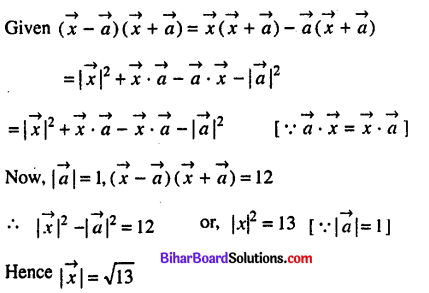Question 17.
Find the distance from the origin to the plane: 2x-3y + 4z – 6 = 0.
Since dr’s of plane is 2, -3,4 then dc’s are :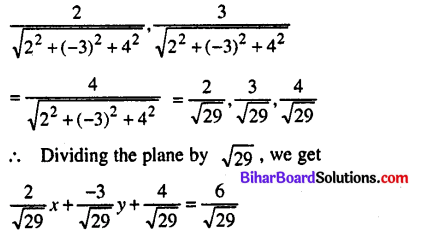Which is in the form of lx + my + nz = d, where d be the distance of the plane from the origin.
Hence the distance of the plane from the origin is $$\frac{6}{\sqrt{29}}$$ units.Question 18.
Find vector equation of a plane which is at a distance of 7 units from the origin and normal to the vector 3î + 5ĵ – 6k̂.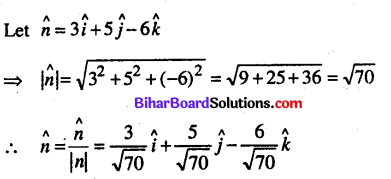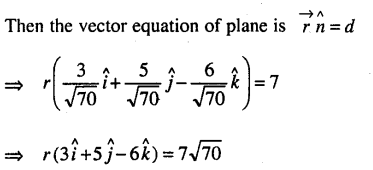Question 19.
Solve : (x + log y )dy + y dx = 0
Given (x + log y )dy + y dx = 0
⇒ (xdy + ydx) + log ydy = 0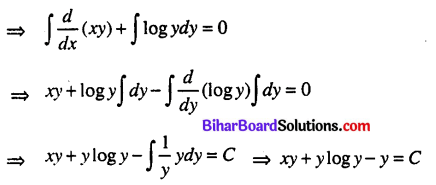Which is the required solution.

Question 20.
Find the slope of tangent to the curve y = x3 – x at x = 2.Question 21.
The probability of an event is 3/7 . Find the odds against the event.
Let A be the any event then P(A) = 3/7
∴ P(A’) = 1 – P(A) = $$1-\frac{3}{7}=\frac{4}{7}$$
Then odds against of the event A = $$\frac{P\left(A^{\prime}\right)}{P(A)}=\frac{\frac{4}{7}}{\frac{3}{7}}$$
= 4/3 = 4:3GCF Calculator is An online calculator to calculate the greatest common factor GCF of two integers.

Question 22.
What is the probability of throwing more than 4 in a single throw from an ordinary die ?
Here n(s) = 6 and 5 and 6 are the greatest number of 4. If A = event of outcome 5 and B = event of outcome 6. Then n(A) = 1 = n(B)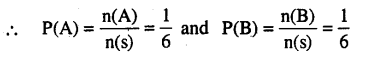Since the outcomes are 5 and 6 are mutually exclusive events due to 5 and 6 not comes together.
The probability of out comes 5 or 6 = P(A∪B)
= P(A) + P(B) = $$\frac{1}{6}+\frac{1}{6}=\frac{1}{3}$$

Question 23.
If the function f:R→R defined as f(x) = x2 – 3x + 2 then find f(f(x)).
Given f:R → R,f(x) = x2 – 3x + 2
∴ f(f(x)) = f(x2 – 3x + 2
= (x2-3x + 2)2 – 3(x2 – 3x + 2) + 2
= (x4 + 9x2 + 4 – 6x3 – 12x + 4x2) + (- 3x2 + 9x – 6) + 2
= x4-6x2 + 10x2 – 3x

Question 24.
Differentiate $$\frac{\cos x}{\log x}$$, x >0 with respect to x.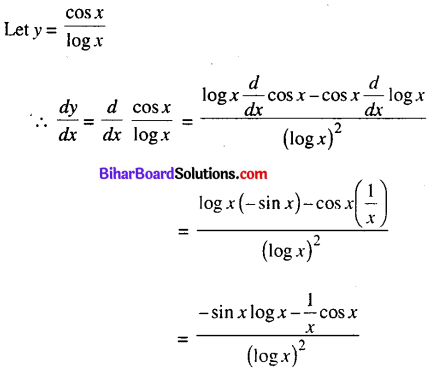Question 25.
Differentiate xsin x with respect to x.
y = xsinx or, logy = logxsin x
or, logy = sin x logx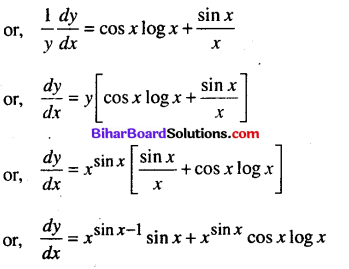Questions No. 26 to 33 are Long Answer Type Questions. Each question carry 5 marks. Answer any 4 question. (4 × 5 = 20)

Question 26.
Find the equation of the curve passing through the point (1,-1) whose differential equation is xy$$\frac{d y}{d x}$$ = (x+2) (y + 2)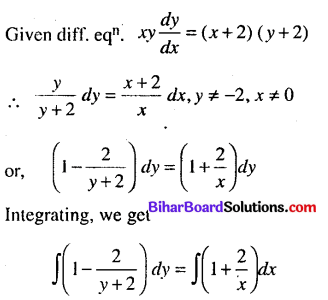or, y – 2log |y + 2| = x + 2log |x| + k ……………1
Since the curve passes through (1,-1), then from (1), we get
-1 – 2log 1 = 1+ 2log 1 + k ∴ k = -2
Hence the equation of the curve is
y – 2log |y + 2| = x + 2log |x| – 2Question 27.
Prove that the maximum value of the function y = $$\left(\frac{1}{x}\right)^{x}$$ is $$e^{1 / e}$$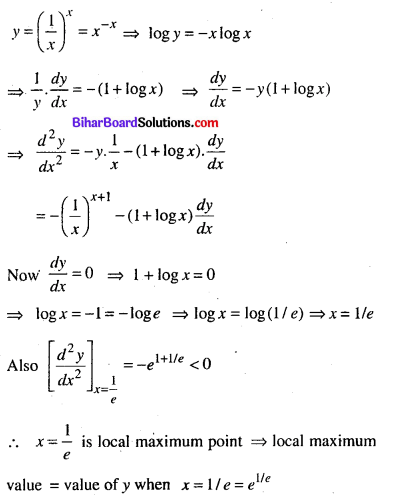Question 28.
Integrate : $$\int \frac{x}{\left(x^{2}+1\right)(x+1)} d x$$Question 29.
Evaluate : $$\int_{0}^{\pi} \frac{x d x}{a^{2} \cos ^{2} x+b^{2} \sin ^{2} x}$$Question 30.
If â and b̂ are two unit vectors and θ be the angle between them then prove that sin θ = $$\frac{1}{2}|\hat{a}-\hat{b}|$$
Since â and b̂ are unit vectors and θ be the angle between them
∴ |â|= 1 ⇒ a2 = 1 or |b̂|= 1 ⇒ b2 = 1
|â – b̂|2 = a2 + b2 – 2abcosθ
|â – b̂|2 = 1 + 1 – 2.1.1cosθ
|â – b̂|2 = 2 – 2cosθ = 2(1 – cosθ) = 2.2sin2Question 31.
If the lines $$\frac{x-1}{-3}=\frac{y-2}{2 k}=\frac{z-3}{2}$$ and $$\frac{x-1}{3 k}=\frac{y-1}{1}=\frac{z-6}{-5}$$ are mutually perpendicular then find the value of k.
Given $$\frac{x-1}{-3}=\frac{y-2}{2 k}=\frac{z-3}{2}$$
and $$\frac{x-1}{3 k}=\frac{y-1}{1}=\frac{z-6}{-5}$$
The dr’s of (1) and (2) are – 3, 2k, 2 and 3k, 1,-5 respectively,
The lines are mutually perpendicular if
a1a2 + b1b2 + c1c2 = 0
or -3(3k) + 2k(1) + 2(-5) = 0
-9k + 2k – 10 = 0 or 7k +10 = 0
k = 10/7Question 32.
Solve the following LPP problem.
Minimize Z = x + 2y
Subject to constraints: 2A +y ≥ 3, x + 2y ≥ 6, x, y ≥ 0.
Minimize Z = A + 2y
under the constraints : 2A + y ≥3 => 2x + y = 3 …(1)
x + 2y ≥ 6 ⇒ x + 2y = 6 .. .(2)
x, y ≥ 0 ⇒ x = 0,y = 0 …(3)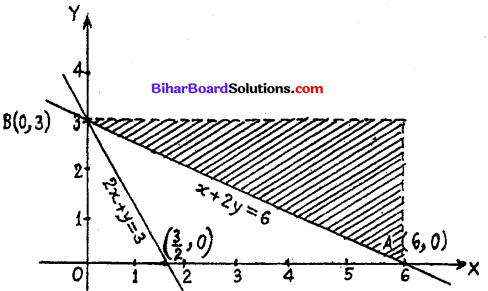First of all draw graph of equations (1) to (3) corresponds to inequations.
It is clear from the figure the line passes through the
points (3/2 , 0) and (0,3).
Putting x = 0, y = 0 in 2x + y ≥ 6, we get 0 ≥ 3.
The region 2x + y ≥ 3 on the line and the part above of line.
Similarly, the line x + 2y = 6 passes through the points A (6,0) and B (0,3).
Putting x = 0, y = 0 in A + 2y ≥ 6, we get 0 ≥ 6.
The region of x + 2y ≥ 6 lie on the line and the part above of line, x ≥ 0, lie on y-axis and the right of y-axis. y ≥ 0, line on x-axis and the above of x-axis.
Hence shaded feasible region XABY is above of line AB.
Now, to find the minimum value of Z = x + 2y from the points A (6,0) and B (0, 3) of the line AB.
Corner Points Z = x + 2y
A(6,0) Z = 6+ 2.0 = 6
A (0,3) Z = 0 + 2.3 = 6
Thus from the above table that the minimum common value of objective function Z is 6 at the both points.Question 33.
A toy company manufactures two types of dolls A and B. Market tests and available resources have indicated that combined production level should not exceed 1200 dolls per week and demand of dolls of type B is at most half of that for dolls of type A. Further, the production level of dolls of type A can exceed three times the production of dolls of other type by at most 600 units. If the company makes profit of Rs. 12 and Rs. 16 per dolls respectively on dolls A and B, how many of each should be produced weekly in order to maximize the profit.
Let the company manufacture of dolls of types A and B are x and y respectively. From the given problem the data are as follows :
x ≥ 0, y ≥ 0 ⇒ x = 0, y = 0 … (i)
x + y ≤ 1200 ⇒ x + y = 1200 …(ii)
y ≤ x/2
⇒ x – 2y ≥ 0 ⇒ x – 2y = 0 … (iii)
x ≤ 3y + 600 ⇒ x – 3y ≤ 600 ⇒ x – 3y = 600 … (iv)
Let the profit from A type of doll is A and B type of doll is y.
∴ Z = 12A + 16y
Now, from (ii) and (iii), we get
im 43
From (iii), we get
A = 2×400 = 800
∴ Co-ordinates of B is (800,400)
Again, from (ii) and (iv), we get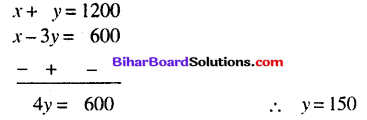From (ii), we get A= 1050
Co-ordinates of A is = (1050,150)
Now, Feasible region = shaded region = ABOC region. Lastly, for maximum profit, to find the value of Z from the co-ordinates of comer point of shaded region.
Corner point Z = 12x + 16y
at (0,0) Z = 0 + 0 = 0
at B (800,400) Z= 12 × 800 + 16 × 400
= 9600 + 6400=16000
at A(1050,150) Z = 12 × 1050 + 16 × 150
= 12600 + 2400 = 15000
From above table, the maximum value of Z is 16000 at the point A = 800, y = 400. Thus 800 dolls of type A and 400 dolls of type be produced and get the maximum profit is Rs. 16000